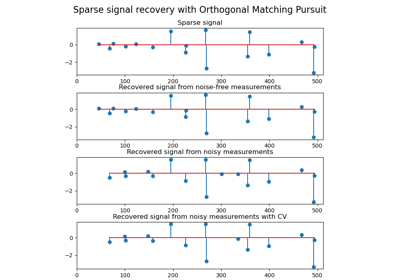# sklearn.datasets.make_sparse_coded_signal¶

sklearn.datasets.make_sparse_coded_signal(n_samples, *, n_components, n_features, n_nonzero_coefs, random_state=None)[source]

Generate a signal as a sparse combination of dictionary elements.

Returns a matrix Y = DX, such as D is (n_features, n_components), X is (n_components, n_samples) and each column of X has exactly n_nonzero_coefs non-zero elements.

Read more in the User Guide.

Parameters
n_samplesint

number of samples to generate

n_componentsint,

number of components in the dictionary

n_featuresint

number of features of the dataset to generate

n_nonzero_coefsint

number of active (non-zero) coefficients in each sample

random_stateint, RandomState instance, default=None

Determines random number generation for dataset creation. Pass an int for reproducible output across multiple function calls. See Glossary.

Returns
dataarray of shape [n_features, n_samples]

The encoded signal (Y).

dictionaryarray of shape [n_features, n_components]

The dictionary with normalized components (D).

codearray of shape [n_components, n_samples]

The sparse code such that each column of this matrix has exactly n_nonzero_coefs non-zero items (X).

## Examples using sklearn.datasets.make_sparse_coded_signal¶